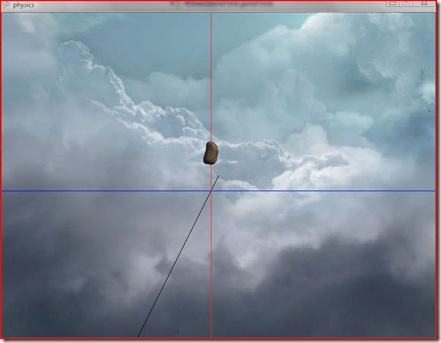## March 11, 2008

### Studying physics with XNA

The past two weeks, I was in holiday, the usual 2-week holiday we have in France a few times a year. I had to study physics for school, and it was almost all about "balistique", which is basically finding the equation of an object's movement once you know its initial state and the forces acting on it, thanks to Newton's second law. Since studying that only on paper wouldn't be fun, I wanted to actually simulate the movements in a 3D environment, to see it more realistically.## Intro to XNA

I had recently discovered XNA, Microsoft's 'new' 3D graphics framework, which makes it a lot easier than DirectX to write 3D games/programs. I've been doing some DirectX previously, and boy I can tell you how cool it is not to care about creating Direct3D devices, pixel formats, present parameters, and all that low-level stuff which is mostly for professional game developers, and focus directly on much higher level things, after Visual Studio generates the your main functions.

The C# programming language is also much better, I think, for 3D. The DirectX programming I did was in C++, and since the C++ DirectX API is (almost)the same one as the C one, it has all those weird naming conventions like the IDirect3D9, D3D, D3DX prefixes to every single interface/class/enum/etc which makes the code unpleasant. In XNA, LPDIRECT3DTEXTURE9 becomes Texture. Loading textures and models is done by simply using the (awesome) Content manager (for example, myTexture = Content.Load<Texture>("asteroid.jpg"); ) instead of using something like D3DXCreateTextureFromFile(g_pDevice, "asteroid.jpg", &m_pMyTexture) (notice the frequent use of double pointers, which is required in every function that creates or loads something). The awesome IntelliSense of Visual Studio is surely a lot more convenient (and VERY useful to even find stuff you didn't know) in C# than it is in C++. I know there's a managed DirectX SDK, but since all my books use C++, I've stick to that language (and by the way, I love C++ when it is used the right way).

So basically what I'm saying is that if you want to do some 3D projects (games or not) just for fun, you should use XNA rather than DirectX. If you want to do game development later then you might want to learn DirectX, but actually, once you know XNA, it should be a lot easier to move to DirectX from there than learning it from scratch.

## The physics "simulator"

Now let's get back to that physics "simulator", and what's in it.

When you create a new XNA project (a "windows game" template), what you have is a Game class, where you have all the main methods such as Initialize(), Update() and Draw().

Then I wrote a 'Solid' class, which represents a physical object, and contains its physical properties (such as its mass or electrical charge) and some other physical quantities used (gravitational, electric and magnetic fields, a friction value etc.). It also has the current parameters of the object, such as its position in space, it's velocity and acceleration (3D vectors); the update() function is where all the physical/mathematical calculations are done, and the Draw() method simply draws the Model in the right place, using the appropriate World, View and Projection matrices.

The view and projection matrices are taken from the Camera class I wrote, which is a static class representing a First Person Camera in space. it basically computes the view matrix from the user input (arrow keys and mouse, as in an FPS game), and it allows you to move around the scene and not to lose the object from your field of view.

Now let's get to the main piece of code that makes the object move:

`   1: //This is where you set the initial state`
`   2: Vector3 position = new Vector3(0.0f, 10.0f, 0.0f);  //Vector3.Zero;`
`   3: Vector3 velocity = Vector3.Zero;   //new Vector3(10.0f, 30.0f, 0.0f);`
`   4: Vector3 acceleration;`
`   5:  `
`   6: public virtual void Update(GameTime gameTime)`
`   7: {`
`   8:     float dt = (float)gameTime.ElapsedGameTime.Milliseconds / 1000;`
`   9:     float t = (float)gameTime.TotalGameTime.Milliseconds / 1000;`
`  10:  `
`  11:     //---------- Newton's 2nd law -------------`
`  12:     `
`  13:     //acceleration = q / m * (E + Vector3.Cross(velocity, B)) - 1.2f * velocity / m;    //electric field + magnetic field + friction`
`  14:     acceleration = (-k / m * position - h * velocity) / m;                              //oscillator with friction`
`  15:     //acceleration = (-k / m * position - h * velocity - 50.0f * (float)Math.Cos(0.01f * t) * Vector3.Up) / m;`
`  16:  `
`  17:     velocity += acceleration * dt;`
`  18:     position += velocity * dt;`
`  19: }`

The dt variable is just the time elapsed between two frames, in seconds (converting to seconds makes things easier for physics calculations). Then you have to write the actual expression of the acceleration from Newton's second law. I put it in the Update() method because sometimes acceleration changes with time (that doesn't happen with gravity alone, but it does as soon of you have friction, for instance).

The two following lines are simply doing the job of integrating over time:

since (vectorially), vel = d(pos)/dt and acc= d(vel)/dt, you have to integrate acceleration over time to get velocity, and integrate velocity to get the position.

So this was basically the piece of code which did everything! I've included three types of movement: the first is a charged particle in an electric field and a magnetic field, the second is a damped oscillation, and the third is a forced oscillation (which doesn't simulate very well yet). To change, just comment out the ones you don't want.

If you're wondering what the equation of that weird first trajectory is... Here it is:

If you wish, I can scan the proof (it's about four pages long, and uses complex numbers =P, but I can do it for you :-)